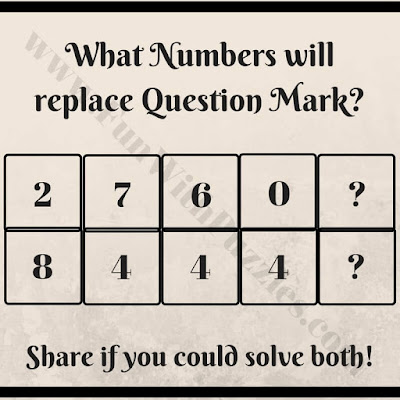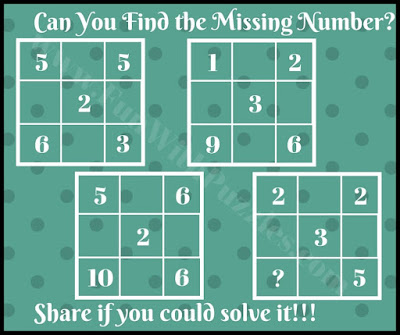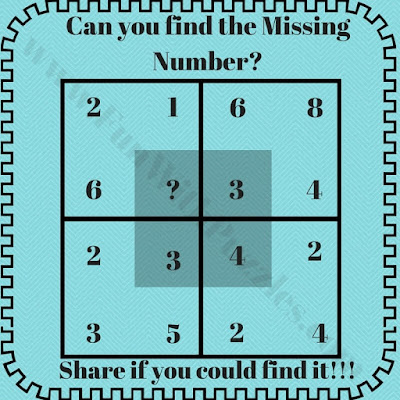## Saturday, December 14, 2019

Here are many different types of puzzles on this website. There are observational questions which will test your visual skills. There are logical questions to test your logical reasoning. There are word questions to test your knowledge of the English language. Also, there are lots of maths questions to test your mathematical skills.
These math questions are of different difficulty level. Some maths questions are very easy which are designed for kids and school-going children. There are some medium difficulty maths questions which are for college studying teens. Also, there are some tough maths questions which are for adults and for job interviews. Today's we are presenting some mind-blowing math questions which are only for Geniuses. These are very tough math puzzles. Let us see how many you can solve it?
In all these mind-blowing questions, a set of few numbers is given around mathematical shape. These numbers are related to each other mathematically or logical. One has to find these relations and then has to find the missing number which will replace the question mark.
So are you ready for this mind-blowing challenge? Answers to these mind-blowing questions are provided as we are looking forward to visitors to solve these questions and post their answers along with an explanation in the comment section. However, if you are not able to answer this question, do worry as links to similar easier questions is provided after each question. Do not forget to check these easier puzzles and post your comment in case you are able to solve any of these mind-blowing questions.1. Mind-bending maths brain picture puzzle challenge2. Mind-blowing math question3. Tough math question4. Tricky math question5. Tricky math question

1.What will be 2nd puzzle ans????
ANd how??

2.What ans in 2nd puzzle??
How?

3.What's the and of 2nd puzzle

4.plz tell me the first puzzle ..mera mind kharab ho gya hai soch soch k

1.Answer to the first puzzle is 3.
Add the top 3 outer numbers and then subtract the 4th lower outer number to get the number in the middle of the Circle.

5.6.